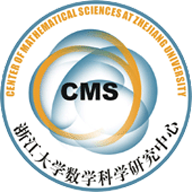(13006) On f-bi-harmonic maps between Riemannian manifolds

Abstract: Both bi-harmonic map and $f$-harmonic map have nice physical motivation and applications. In this paper, by combination of these two harmonic maps, we introduce and study $f$-bi-harmonic maps as the critical points of the $f$-bi-energy functional $\frac{1}{2}\int_M f|\tau (\phi)|^2dv_{g}$. This class of maps generalizes both concepts of harmonic maps and bi-harmonic maps. We first derive the $f$-biharmonic map equation and then use it to study $f$-bi-harmonicity of some special maps, including conformal maps between  manifolds of same dimensions, some product maps between direct product manifold and singly warped product manifold, some projection maps from and some inclusion maps into a warped product manifold.

• f-bi-harmonic 2013-5-23.pdf